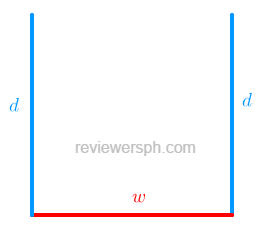### Math Notes

Subjects

#### Algebra Solutions

##### Topics || Problems

An open rectangular gutter is to be made by turning up the sides of a piece of metal 18 inches wide. If the cross-section area of the resulting gutter is 36 square inches, find the gutter's depth.Perimeter of the gutter $$P = 2d +w = 18$$

Area of the gutter: $$A = dw = 36$$

$$w = \frac{36}{d}$$

$$P = 2d + \frac{36}{d} = 18$$

$$18d =2d^2 +36$$

$$d^2 - 9d + 18 =0$$

$$d_1 = \frac{9 +\sqrt{9^2-4(18)}}{2}$$

$$d_1 = 6 ~inches$$

$$d_2 = \frac{9 -\sqrt{9^2-4(18)}}{2}$$

$$d_2 =3 ~inches$$

Check what value is correct (answer).

$$w = \frac{36}{6} = 6$$

$$w = \frac{36}{3} = 12$$

$$A = 12x3 = 6x6 = 36$$ and $$P = 2(3) + 12 = 2(6) +6 = 18$$

Since both 6" and 3" gives a correct area and perimeter the answer is either $$3 ~inches$$ or $$6 ~inches$$